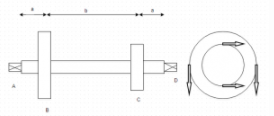Courses

# Test: Shaft Design

## 15 Questions MCQ Test Machine Design | Test: Shaft Design

Description
This mock test of Test: Shaft Design for Mechanical Engineering helps you for every Mechanical Engineering entrance exam. This contains 15 Multiple Choice Questions for Mechanical Engineering Test: Shaft Design (mcq) to study with solutions a complete question bank. The solved questions answers in this Test: Shaft Design quiz give you a good mix of easy questions and tough questions. Mechanical Engineering students definitely take this Test: Shaft Design exercise for a better result in the exam. You can find other Test: Shaft Design extra questions, long questions & short questions for Mechanical Engineering on EduRev as well by searching above.
QUESTION: 1

### While designing shaft on the basis of torsional rigidity, angle of twist is given by?

Solution:

Explanation: θ=(180/π)xMl/GJ where J=πd⁴/32.

QUESTION: 2

### According to ASME code, maximum allowable shear stress is taken as X% of yield strength or Y% of ultimate strength.

Solution:

Explanation: ASME Standard. The lesser value is taken among the two.

QUESTION: 3

### Does ASME Standard take into consideration shock and fatigue factors?

Solution:

Explanation: Moment is multiplied by a number to consider these factors while designing the shaft.

QUESTION: 4The layout of a shaft supported on bearings at A & B is shown. Power is supplied by means of a vertical belt on pulley B which is then transmitted to pulley C carrying a horizontal belt. The angle of wrap is 180’ and coefficient of friction is 0.3. Maximum permissible tension in the rope is 3kN. The radius of pulley at B & C is 300mm and 150mm.

Q. Calculate the torque supplied to the shaft.

Solution:

Explanation: M=(P₁-P₂)xR where P₁=3000N, P₂=P₁/e^(Coeff of friction x Angle of wrap).

QUESTION: 5The layout of a shaft supported on bearings at A & B is shown. Power is supplied by means of a vertical belt on pulley B which is then transmitted to pulley C carrying a horizontal belt. The angle of wrap is 180’ and coefficient of friction is 0.3. Maximum permissible tension in the rope is 3kN. The radius of pulley at B & C is 300mm and 150mm.

Q. Calculate the tension in the rope of pulley C.

Solution:

Explanation: (P₃-P₄)x150=549.3×1000 and P₃/P₄=2.6. Hence P₃=5948.15N and P₄=2288.75N.

QUESTION: 6The layout of a shaft supported on bearings at A & B is shown. Power is supplied by means of a vertical belt on pulley B which is then transmitted to pulley C carrying a horizontal belt. The angle of wrap is 180’ and coefficient of friction is 0.3. Maximum permissible tension in the rope is 3kN. The radius of pulley at B & C is 300mm and 150mm.

Q. If allowable shear stress in the shaft is 70N/mm² and torsional and bending moments are M=1185000N-mm and m=330000N-mm, find the diameter of the shaft.

Solution:

Explanation: 70=(16/πdᵌ)x√M²+m².

QUESTION: 7The layout of a shaft supported on bearings at A & B is shown. Power is supplied by means of a vertical belt on pulley B which is then transmitted to pulley C carrying a horizontal belt. The angle of wrap is 180’ and coefficient of friction is 0.3. Maximum permissible tension in the rope is 3kN. The radius of pulley at B & C is 300mm and 150mm.

Q.  If bending moment on point B in horizontal plate is M and in vertical plane is m, then the net bending moment at point B is?

Solution:

Explanation: The two moments act perpendicularly to each other.

QUESTION: 8

Calculate the shaft diameter on rigidity basis if torsional moment is 196000N-mm, length of shaft is 1000mm. Permissible angle of twist per meter is 0.5’ and take G=79300N/mm².

Solution:

Explanation: d⁴=584Ml/Gθ.

QUESTION: 9

If yielding strength=400N/mm², the find the permissible shear stress according to ASME standards.

Solution:

Explanation: 0.18×400.

QUESTION: 10

The stiffness of solid shaft is more than the stiffness of hollow shaft with same weight.

Solution:

Explanation: Hollow shaft is more stiff.

QUESTION: 11

The strength of hollow shaft is more than the strength of solid shaft of same weight.

Solution:

Explanation: Outer fibers are more effective in resisting the applied moments. In hollow shafts material is removed and spread on a larger radius.

QUESTION: 12

Solid shaft is costlier than hollow shaft of same weight.

Solution:

Explanation: Hollow shaft cost is more as material is to be selectively emplaced.

QUESTION: 13

Solid shafts are used in epicyclic gearboxes.

Solution:

Explanation: In epicyclic gears, one shaft rotates inside other and hence hollow shafts are used.

QUESTION: 14

Flexible shafts have ___ rigidity in torsion making them flexible.

Solution:

Explanation: Flexible shafts have high rigidity in torsion making then capable to transmit torque.

QUESTION: 15

Flexible shafts have ______ rigidity in bending moment.

Solution:

Explanation: Flexible shafts have low rigidity in bending moments making them flexible.